Introduction: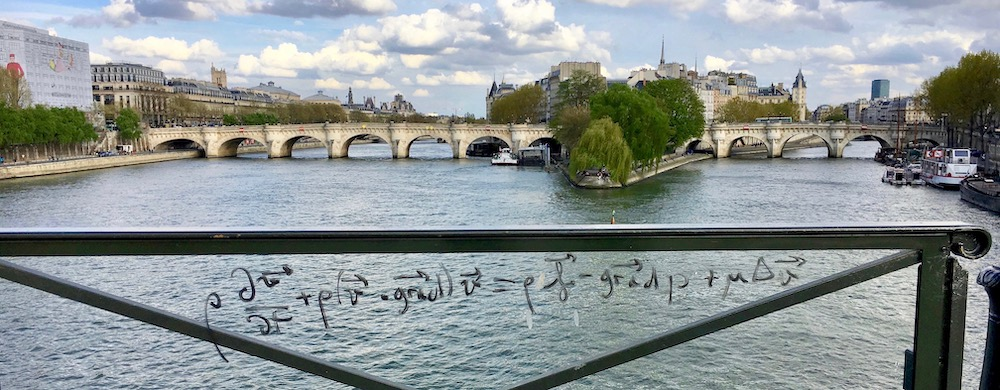In the following page you'll find materials for course Advanced Hydrodynamics taught at Warsaw University Physics Department in 2021.

This course follows problem-based learning and materials are divided into modules related to specific experiments involving flow.

In many cases we'll use Mathematica to quickly test different models during discussions. Slightly cleaned up notebooks will be uploaded after meets

Mathematica licences can be obtained here.

1. Vacuum bazooka. An introduction: drag coefficient, Reynolds number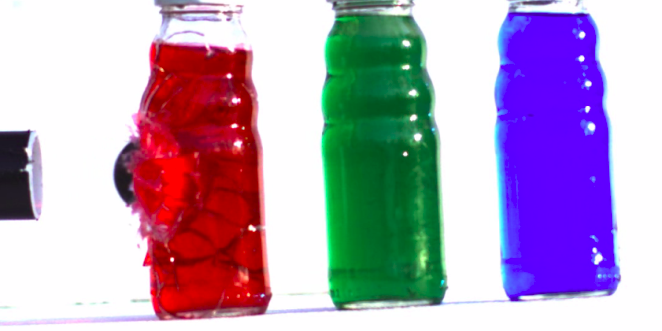Following prompt was given during International Young Physicist Torunament:

A ‘vacuum bazooka’ can be built with a simple plastic pipe, a light projectile, and a vacuum cleaner. Build such a device and maximise the muzzle velocity.

You can find an example of such device in this YouTube video or this video.

How would one optimize such device? How does the bullet interact with the flow? What physical phenomena limit muzzle velocity?

A Mathematica notebook (stub) for optimizing bazookas: link.

2. Leidenfrost stars. Potential flow, capilary waves.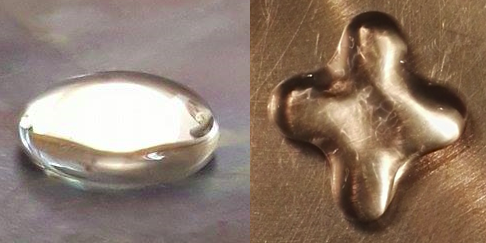Following prompt was given during International Young Physicist Torunament:

In the Leidenfrost effect, a water drop placed on a hot surface can survive for minutes. Under certain circumstances, such a drop develops oscillating star shapes. Induce different oscillatory modes and investigate them.

It's quite easy to recreate in your home (try it!), but you can also look at a slow motion vide here for example.

Why do they vibrate? What is the role of the Leidenfrost effect? How to find the period of oscillation?

More depth:

Questions to the Phys.Rev.Fluids paper:

• What are the determinants for selection of a particular mode of oscillations?
• What can you say about wavelengths of the capillary waves under the drop? Which dimension of the drop determines them?
• What is the mechanism that causes star shaped oscillations? What reasoning lead authors to such a conclusion?
• What are relevant length scales in this phenomenon? Why do authors prefer one to the other when calculating Reynolds numbers?
• How are relevant quantities (velocity, pressure, temperature) distributed below the droplet? What causes such distribution?
• What characterizes experimental setup used by the authors? How is it different/similar to experiment done in the kitchen?

3. Filling up a Bottle. Quasi 1D approximations.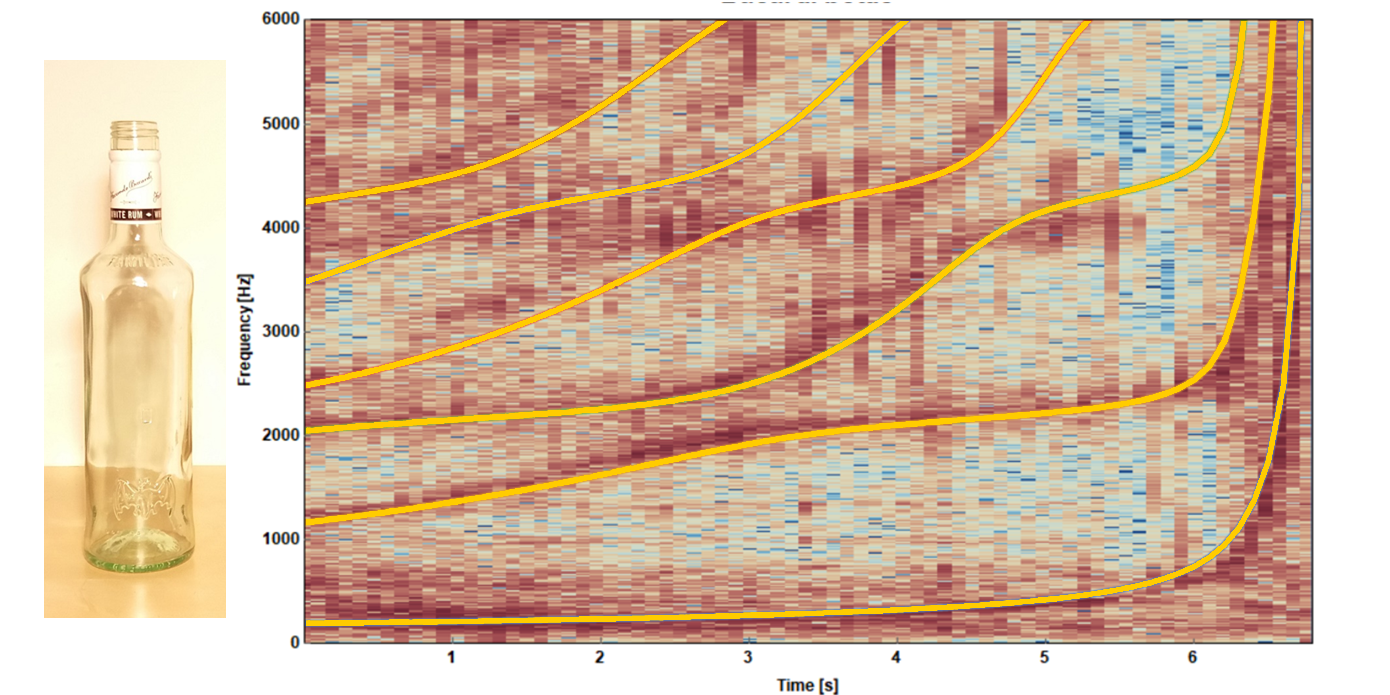Following prompt was given during International Young Physicist Torunament:

When a vertical water jet enters a bottle, sound may be produced, and, as the bottle is filled up, the properties of the sound may change. How does the bottle size and shape influence the phenomenon?

If you need a video reference try here.

Compare the value of dominant frequency observed to acoustic resonance of half-open cyllinder. What did you find?

4. Hydraulic jump. Froude number.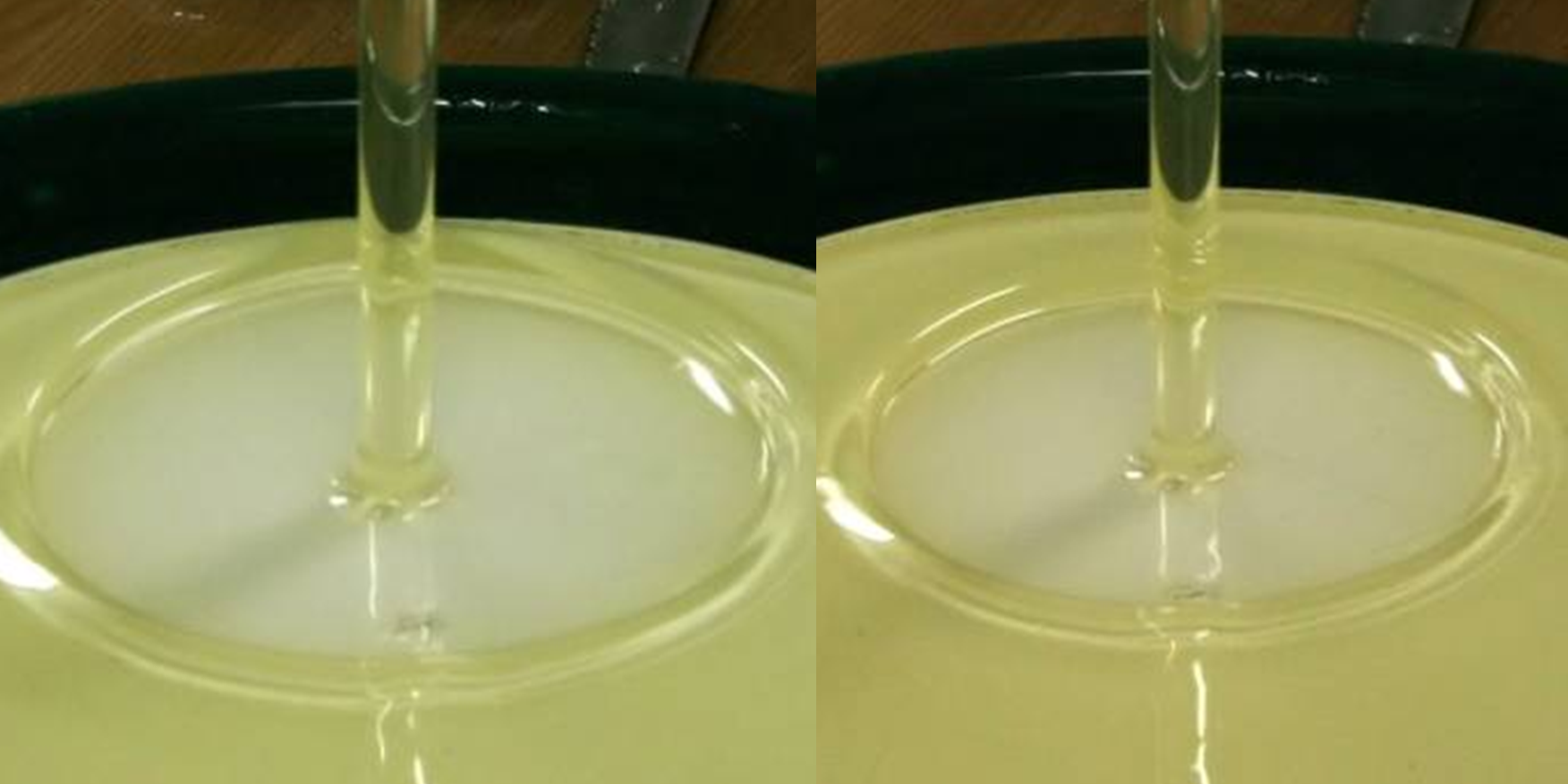Following prompt was given during International Young Physicist Torunament:

When a smooth column of water hits a horizontal plane, it flows out radially. At some radius, its height suddenly rises. Investigate the nature of the phenomenon. What happens if a liquid more viscous than water is used?

You can find demonstration of a hydraulic jump in a clear plastic setup here.

Use a flat plane (such as cutting board, most plates are too concave) below a faucet to observe hydraulic jump a different velocities of the impinging jet. What can you say about the radius of the jump?

More depth:

Questions to the J.Fluid.Mech. paper:

• What is the role of gravity in this problem?
• What is the role of surface tension in this problem?
• What properties of the fluid are relevant?
• If we scale the setup by a factor of 10 what would happen to the jump radius? How would it scale?

Half way assignment. Instability of a liquid jet

Produce a stable laminar jet of flowing water by opening the kitchen tap (or otherwise). After travelling some length, an instability develops and the jet breaks up into droplets. Investigate this phenomenon. Explore the shape of the falling jet, the breakup mechanism and the evolution of the stream. An analogous phenomenon happens with a liquid sheet, e.g. one flowing out from a square-shaped box over the side. Discuss the similarities and differences.

For the observations, you can use plotting paper and/or a mobile phone with a camera (and possibly a slow motion video mode).
Pay particular attention to include the following parts in your mini-paper:

• Introduction – with a literature review of published papers on the problem. (Use tools like arxiv and google scholar, read abstracts and select some publications you think are relevant. Pay particular attention to discussed phenomena, theoretical assumptions and explanatory power when quoting previous work).
• Qualitative presentation of the phenomenon – important parameters, dimensionless numbers, what physical mechanisms shape the flow. (Detailed discussions are welcome, but an overall concise explanation is essential. Preferably, it should be understandable to non-experts).
• Theoretical model used to describe the observations – quantification of previously introduced notions. (Pick something that you really observe in the toy experiment you made. Try working with very simple models first, especially if you can comment on the ranges of applicability of them).
• Comparison of theoretical results and experimental observations – (Discuss differences between the two, where do they come from: experimental setup or theoretical limitations? How do they compare to measurements you can find in the literature?)
• Conclusions. – (Sell what you’ve done. Bring attention of the reader to the parts you’re most satisfied with).

TBA